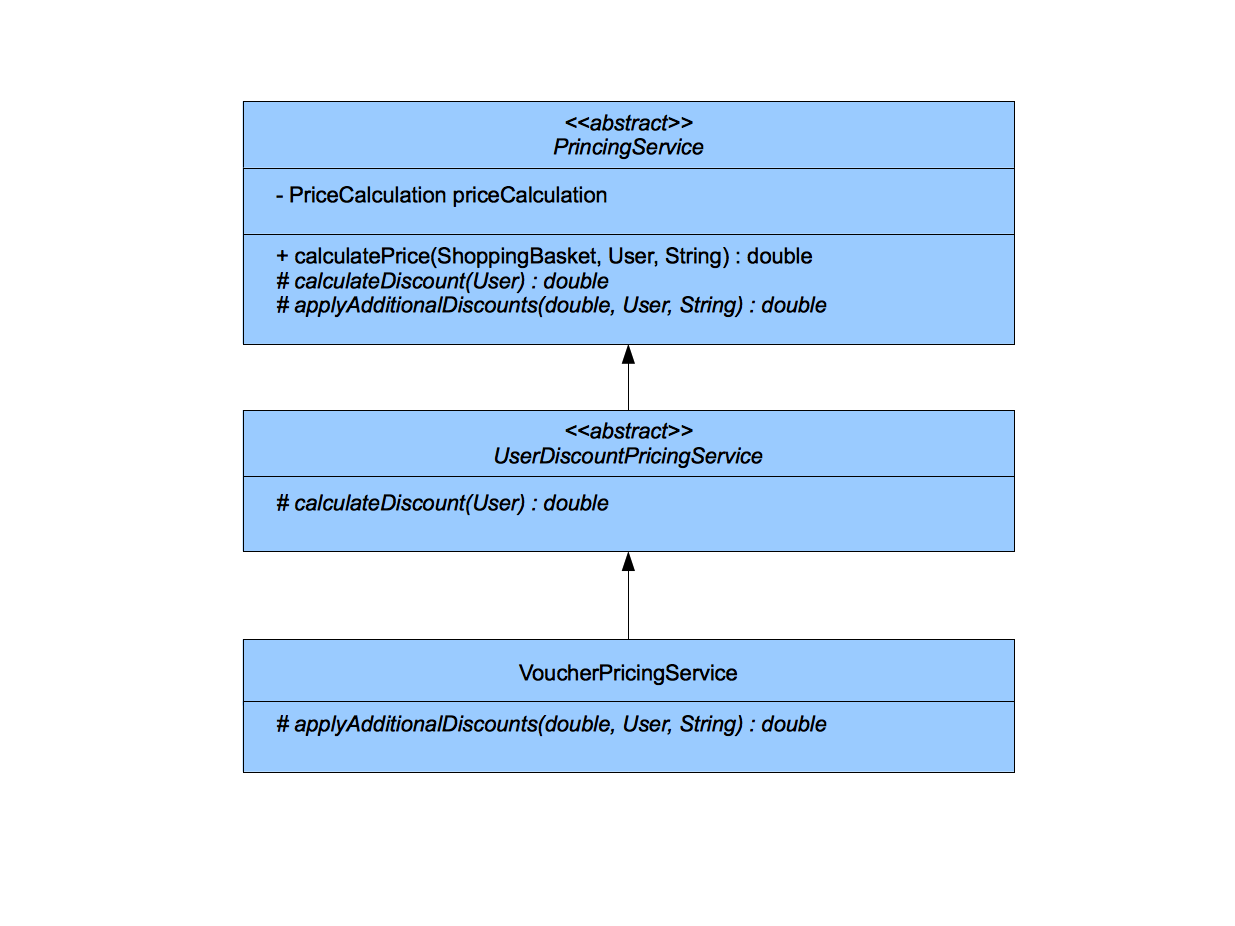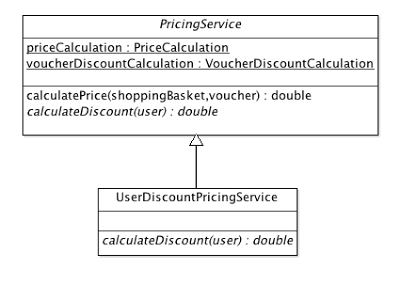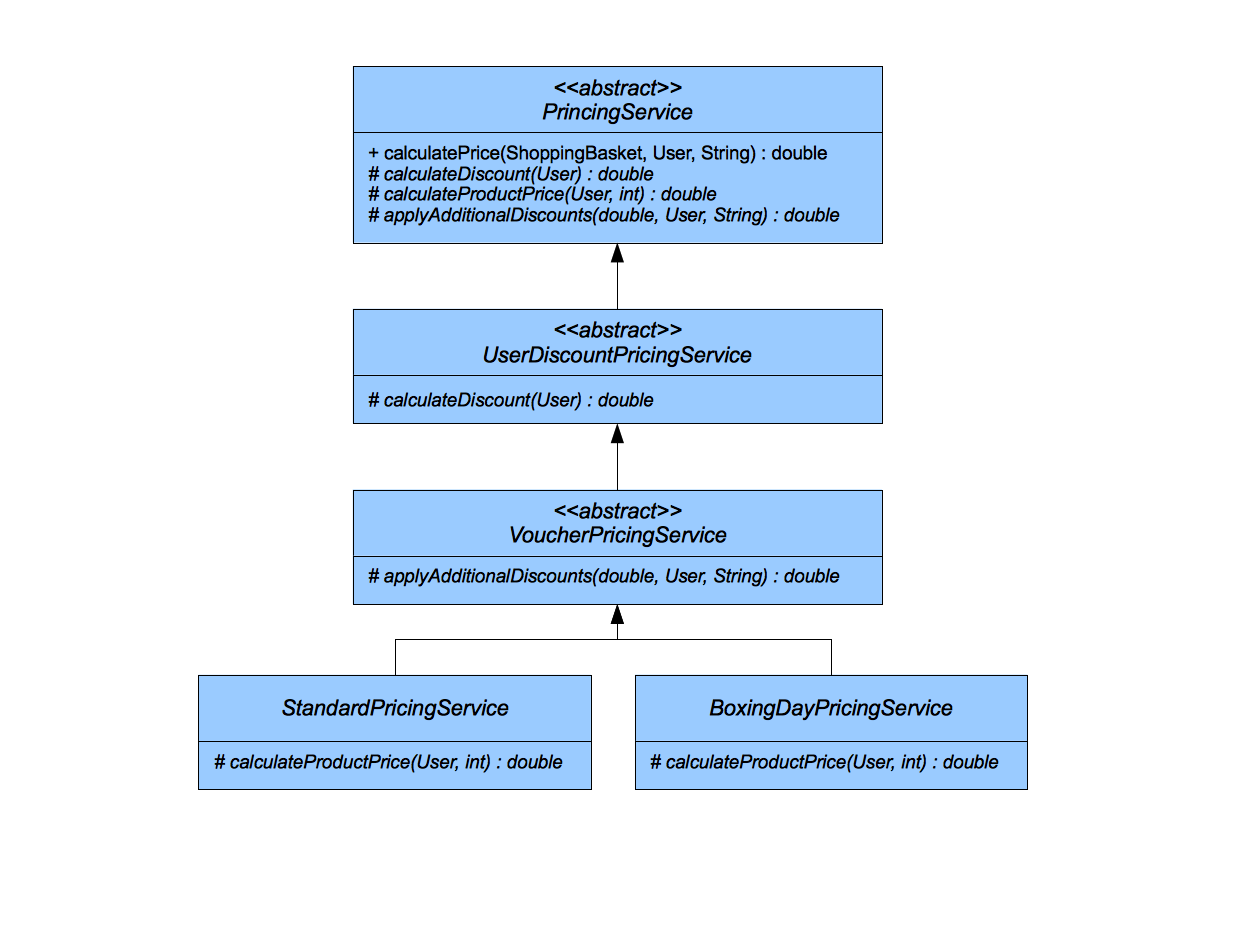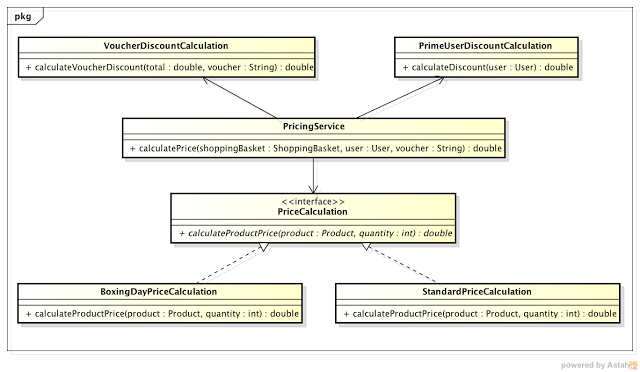# Extract, Inject, Kill: Breaking hierarchies (part 3)

26 Mar 2012## Sandro Mancuso

See author's bio and posts

In part one I explain the main idea behind this approach and in part two I start this example. Please read parts one and two before reading this post

Although the main ideas of Extract, Inject, Kill is already expressed, it's good to finish the exercise just for completion's sake. Here is where we stopped:Let's have a look at the VoucherPricingService, that now is the only concrete class at the bottom of our hierarchy.

``````public class VoucherPricingService extends UserDiscountPricingService {

private VoucherService voucherService;

@Override
protected double applyAdditionalDiscounts(double total, User user, String voucher) {
double voucherValue = voucherService.getVoucherValue(voucher);
double totalAfterValue = total - voucherValue;
return (totalAfterValue > 0) ? totalAfterValue : 0;
}

public void setVoucherService(VoucherService voucherService) {
this.voucherService = voucherService;
}
}
``````

Note that it uses the VoucherService class to calculate the voucher value.

``````public class VoucherService {
public double getVoucherValue(String voucher) {
// Imagine that this calculate the voucher price.
// Keeping it simple so we can understand the approach.
return 0;
}
}
``````

Before anything, let's write some tests to VoucherPricingService.java

``````@RunWith(MockitoJUnitRunner.class)
public class VoucherPricingServiceTest {

private static final User UNUSED_USER = null;
private static final String NO_VOUCHER = null;
private static final String TWENTY_POUNDS_VOUCHER = "20";

@Mock private VoucherService voucherService;
private TestableVoucherPricingService voucherPricingService;

@Before
public void initialise() {
voucherPricingService = new TestableVoucherPricingService();
voucherPricingService.setVoucherService(voucherService);
when(voucherService.getVoucherValue(TWENTY_POUNDS_VOUCHER)).thenReturn(20D);
}

double returnedAmount = voucherPricingService.applyAdditionalDiscounts(1000, UNUSED_USER, NO_VOUCHER);

assertThat(returnedAmount, is(1000D));
}

@Test public void should_subtract_voucher_value_from_total() {
double returnedAmount = voucherPricingService.applyAdditionalDiscounts(30D, UNUSED_USER, TWENTY_POUNDS_VOUCHER);

assertThat(returnedAmount, is(equalTo(10D)));
}

@Test public void shoudl_return_zero_if_voucher_value_is_higher_than_total() {
double returnedAmount = voucherPricingService.applyAdditionalDiscounts(10D, UNUSED_USER, TWENTY_POUNDS_VOUCHER);

assertThat(returnedAmount, is(equalTo(0D)));
}

private class TestableVoucherPricingService extends VoucherPricingService {

@Override
protected double applyAdditionalDiscounts(double total, User user, String voucher) {
}
}
}
``````

Once thing to notice is that the User parameter is not used for anything. So let's remove it.

Now it is time to user the Extract, Inject, Kill on the VoucherPricingService. Let's Extract the content of the VoucherPricingService.applyAdditionalDiscounts(double, String) method and add it to a class called VoucherDiscountCalculation. Let's call the method calculateVoucherDiscount(). Of course, let's do that writing our tests first. They need to test exactly the same things that are tested on VoucherPricingService.applyAdditionalDiscounts(double, String). We also take the opportunity to pass the VoucherService object into the constructor of VoucherDiscountCalculation.

``````@RunWith(MockitoJUnitRunner.class)
public class VoucherDiscountCalculationTest {

private static final String NO_VOUCHER = null;
private static final String TWENTY_POUNDS_VOUCHER = "20";

@Mock
private VoucherService voucherService;
private VoucherDiscountCalculation voucherDiscountCalculation;

@Before
public void initialise() {
voucherDiscountCalculation = new VoucherDiscountCalculation(voucherService);
when(voucherService.getVoucherValue(TWENTY_POUNDS_VOUCHER)).thenReturn(20D);
}

double returnedAmount = voucherDiscountCalculation.calculateVoucherDiscount(1000, NO_VOUCHER);

assertThat(returnedAmount, is(1000D));
}

@Test public void should_subtract_voucher_value_from_total() {
double returnedAmount = voucherDiscountCalculation.calculateVoucherDiscount(30D, TWENTY_POUNDS_VOUCHER);

assertThat(returnedAmount, is(equalTo(10D)));
}

@Test public void should_return_zero_if_voucher_value_is_higher_than_total() {
double returnedAmount = voucherDiscountCalculation.calculateVoucherDiscount(10D, TWENTY_POUNDS_VOUCHER);

assertThat(returnedAmount, is(equalTo(0D)));
}

}
``````
``````public class VoucherDiscountCalculation {
private VoucherService voucherService;

public VoucherDiscountCalculation(VoucherService voucherService) {
this.voucherService = voucherService;
}

public double calculateVoucherDiscount(double total, String voucher) {
double voucherValue = voucherService.getVoucherValue(voucher);
double totalAfterValue = total - voucherValue;
return (totalAfterValue > 0) ? totalAfterValue : 0;
}
}
``````

If you noticed, when doing the extraction, we took the opportunity to give proper names to our new classes and methods and also to pass their essential dependencies to the constructor instead of using method injection.   Let's now change the code in the VoucherPricingServiceto use the new VoucherDiscountCalculation and see if all the tests still pass.

``````public class VoucherPricingService extends UserDiscountPricingService {

private VoucherService voucherService;

@Override
protected double applyAdditionalDiscounts(double total, String voucher) {
VoucherDiscountCalculation voucherDiscountCalculation = new VoucherDiscountCalculation(voucherService);
return voucherDiscountCalculation.calculateVoucherDiscount(total, voucher);
}

public void setVoucherService(VoucherService voucherService) {
this.voucherService = voucherService;
}
}
``````

Cool. All the tests still pass, meaning that we have the same behaviour, but now in the VoucherDiscountCalculation class, and we are ready to move to the Inject stage.

Let's now inject VoucherDiscountCalculation into PricingService, that is the top class in the hierarchy. As always, let's add a test that will test this new collaboration.

``````@RunWith(MockitoJUnitRunner.class)
public class PricingServiceTest {

private static final String NO_VOUCHER = "";
private static final String FIVE_POUNDS_VOUCHER = "5";

private TestablePricingService pricingService = new TestablePricingService();

@Mock private PriceCalculation priceCalculation;
@Mock private VoucherDiscountCalculation voucherDiscountCalculation;

@Before
public void initialise() {
this.pricingService.setPriceCalculation(priceCalculation);
this.pricingService.setVoucherDiscountCalculation(voucherDiscountCalculation);
}

@Test public void should_calculate_price_of_all_products() {
Product book = aProduct().named("book").costing(10).build();
Product kindle = aProduct().named("kindle").costing(80).build();
.with(2, book)
.with(3, kindle)
.build();

double price = pricingService.calculatePrice(shoppingBasket, new User(), NO_VOUCHER);

verify(priceCalculation, times(1)).calculateProductPrice(book, 2);
verify(priceCalculation, times(1)).calculateProductPrice(kindle, 3);
}

@Test public void should_calculate_voucher_discount() {
Product book = aProduct().named("book").costing(10).build();
when(priceCalculation.calculateProductPrice(book, 2)).thenReturn(20D);
.with(2, book)
.build();

double price = pricingService.calculatePrice(shoppingBasket, new User(), FIVE_POUNDS_VOUCHER);

verify(voucherDiscountCalculation, times(1)).calculateVoucherDiscount(20, FIVE_POUNDS_VOUCHER);
}

private class TestablePricingService extends PricingService {

@Override
protected double calculateDiscount(User user) {
return 0;
}

@Override
protected double applyAdditionalDiscounts(double total, String voucher) {
return 0;
}

}
}
``````

And here is the changed PriningService.

``````public abstract class PricingService {

private PriceCalculation priceCalculation;
private VoucherDiscountCalculation voucherDiscountCalculation;

double discount = calculateDiscount(user);
double total = 0;
total += priceCalculation.calculateProductPrice(item.getProduct(), item.getQuantity());
}
total = voucherDiscountCalculation.calculateVoucherDiscount(total, voucher);
}

protected abstract double calculateDiscount(User user);

protected abstract double applyAdditionalDiscounts(double total, String voucher);

public void setPriceCalculation(PriceCalculation priceCalculation) {
this.priceCalculation = priceCalculation;
}

public void setVoucherDiscountCalculation(VoucherDiscountCalculation voucherDiscountCalculation) {
this.voucherDiscountCalculation = voucherDiscountCalculation;
}

}
``````

Now it is time to kill the VoucherPricingService class and kill the PricingService.applyAdditionalDiscounts(double total, String voucher) template method, since it is not being used anymore. We can also kill the VoucherPricingServiceTest class and fix the PricingServiceTest removing the applyAdditionalDiscounts() method from the testable class.

So now, of course, we don't have a concrete class in our hierarchy anymore, since the VoucherPricingService was the only one. We can now safely promote UserDiscountPricingService to concrete.

That is now how our object graph looks like:

``````public class PricingService {

private PriceCalculation priceCalculation;
private VoucherDiscountCalculation voucherDiscountCalculation;
private PrimeUserDiscountCalculation primeUserDiscountCalculation;

public PricingService(PriceCalculation priceCalculation, VoucherDiscountCalculation voucherDiscountCalculation, PrimeUserDiscountCalculation primeUserDiscountCalculation) {
this.priceCalculation = priceCalculation;
this.voucherDiscountCalculation = voucherDiscountCalculation;
this.primeUserDiscountCalculation = primeUserDiscountCalculation;
}

total = applyVoucherDiscount(voucher, total);
}

private double userDiscount(User user) {
return primeUserDiscountCalculation.calculateDiscount(user);
}

private double applyVoucherDiscount(String voucher, double total) {
return voucherDiscountCalculation.calculateVoucherDiscount(total, voucher);
}

private double totalAfterUserDiscount(double total, double discount) {
}

double total = 0;
total += priceCalculation.calculateProductPrice(item.getProduct(), item.getQuantity());
}
}

}
``````Our hierarchy is another level short. The only thing we need to do now is to apply  Extract, Inject, Kill once again, extracting the logic inside UserDiscountPricingService into another class (e.g. UserDiscountCalculation), inject UserDiscountCalculation into PricingService, finally kill UserDiscountPricingService and the calculateDiscount(User user) template method.  UserDiscountPricingService,

Since the approach was described before, there is no need to go step by step anymore. Let's have a look at the final result.

Here is the diagram representing where we started:After the last Extract, Inject, Kill refactoring, this is what we've got:The cool thing about the final model pictured above is that now we don't have any abstract classes anymore. All classes and methods are concrete and every single class is independently testable.

That's how the final PricingService class looks like:

For a full implementation of the final code, please look at https://github.com/sandromancuso/breaking-hierarchies

Note: For this three part blog post I used three different approaches to drawing UML diagrams. By hand, using ArgoUML and Astah community edition. I'm very happy with the latter.